# Maharashtra (MSBSHSE) SSC Board Question Paper For Class 10 Maths Paper 1 (Geometry) 2018 In PDF

## Maharashtra SSC Maths Geometry (Paper 1) Exam Question Paper 2018 with Solutions – Free Download

MSBSHSE (Maharashtra board) Public Exam Class 10 Maths 2018 Geometry Question Paper with solutions are given at BYJU’S in a pdf format, which can be downloaded at any time. MSBSHSE has two Maths question papers based on the topics available in the syllabus of MSBSHSE Class 10 Maths textbook. One paper contains Geometry concepts covered and another contains Algebra concepts. Hence, students should focus on both the categories to score maximum marks in the annual examination. Students can also get all the previous years’ SSC board question papers of Maharashtra Class 10 at BYJU’s. These SSC question papers will help the students in the preparation for their final exam to practise different types of problems.

### MSBSHSE Class 10 Mathematics Question Paper 2018 Geometry Paper with Solutions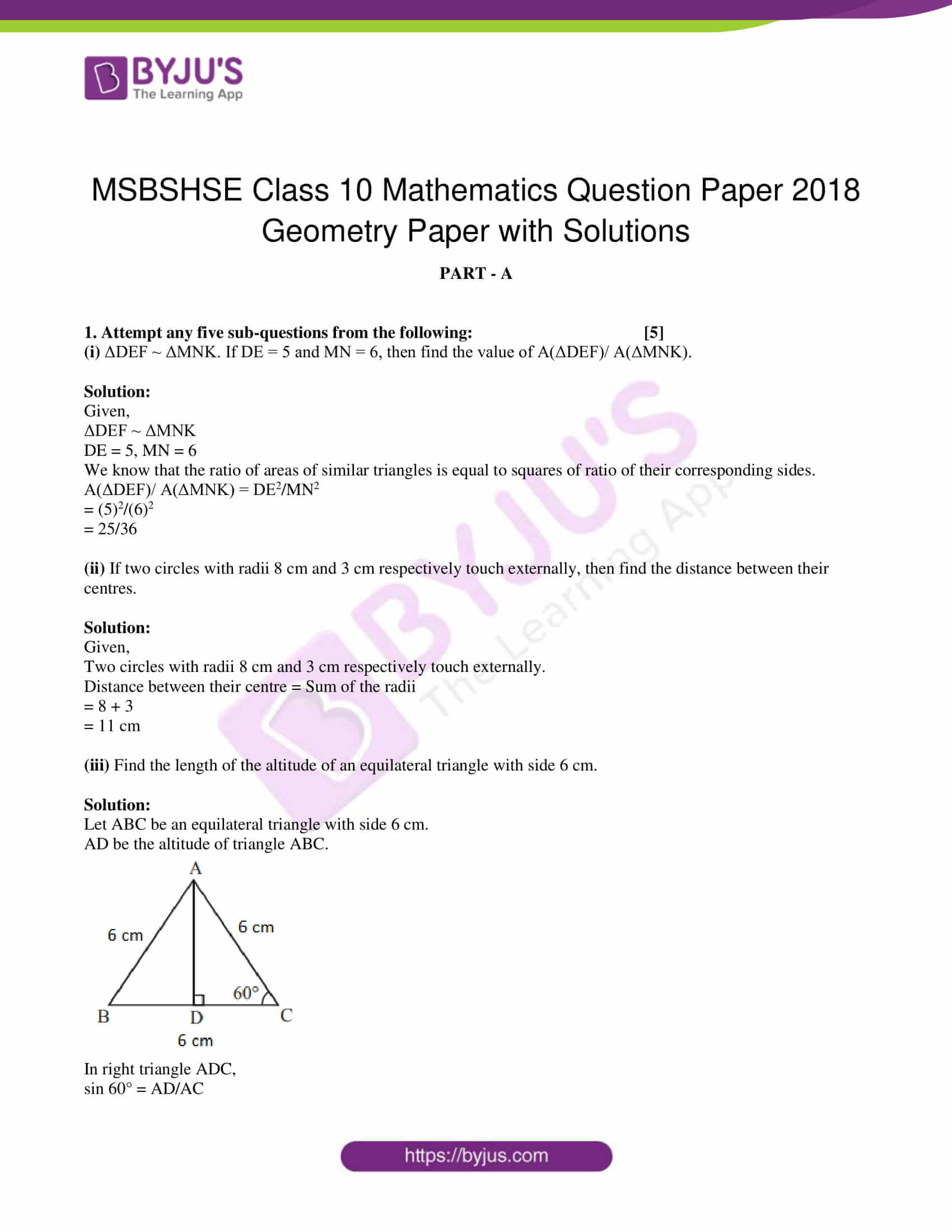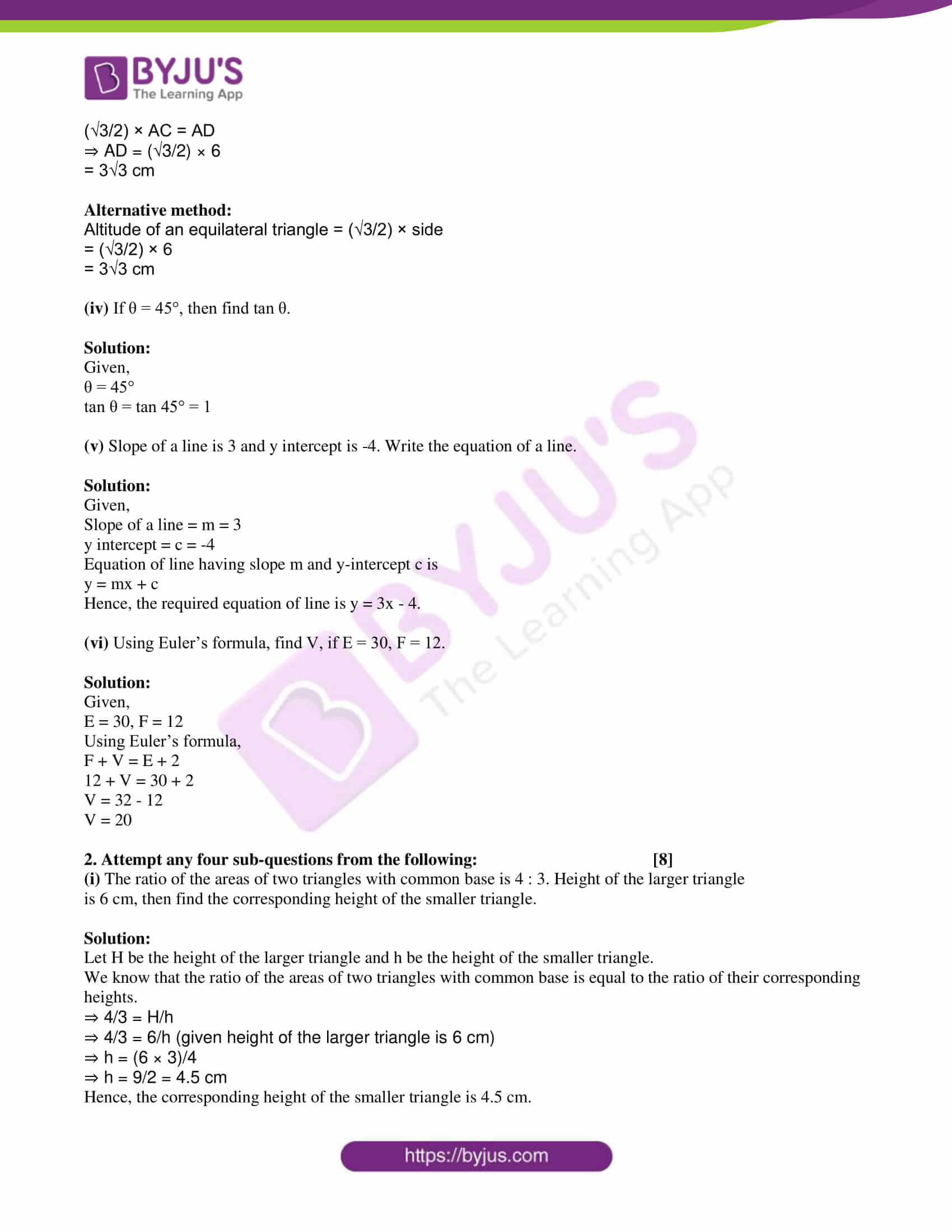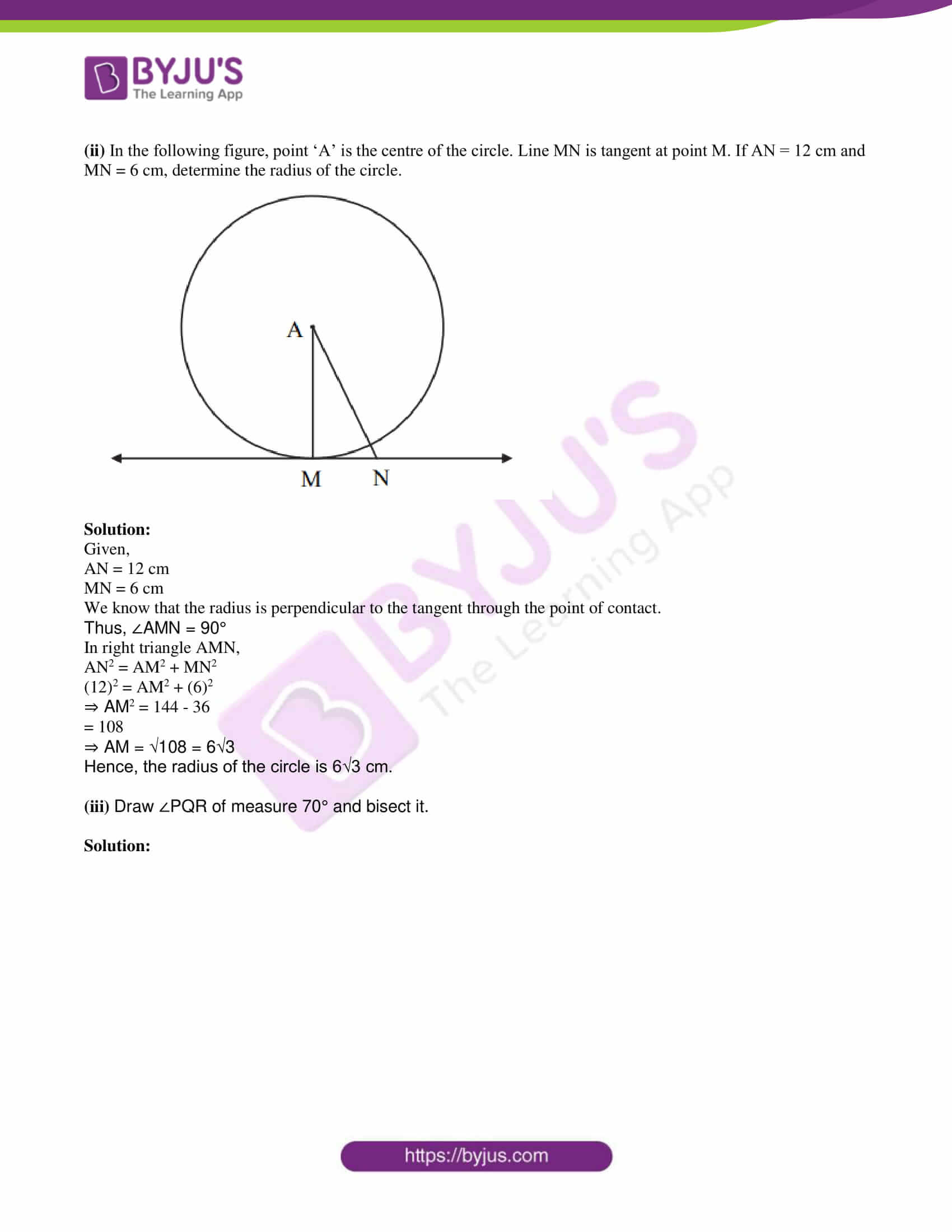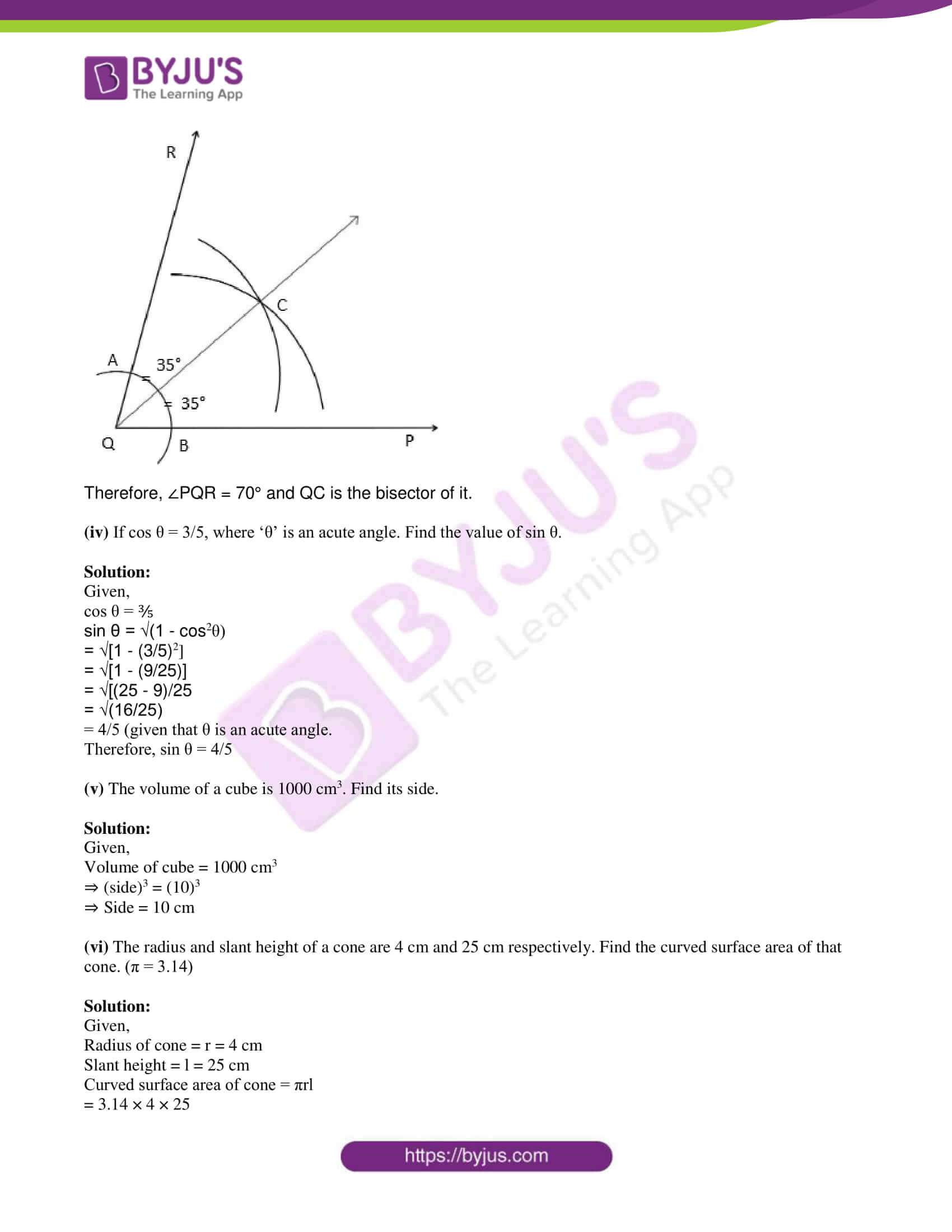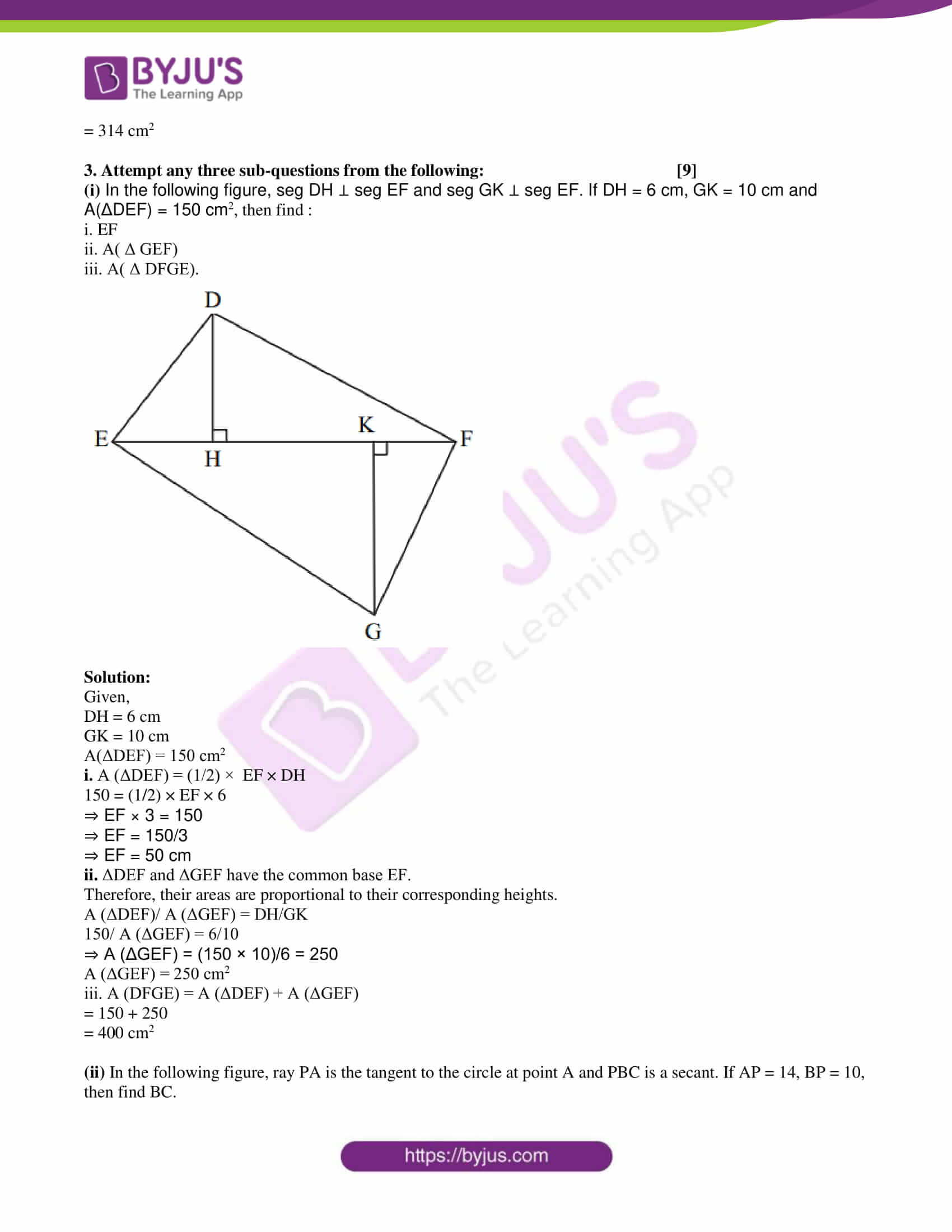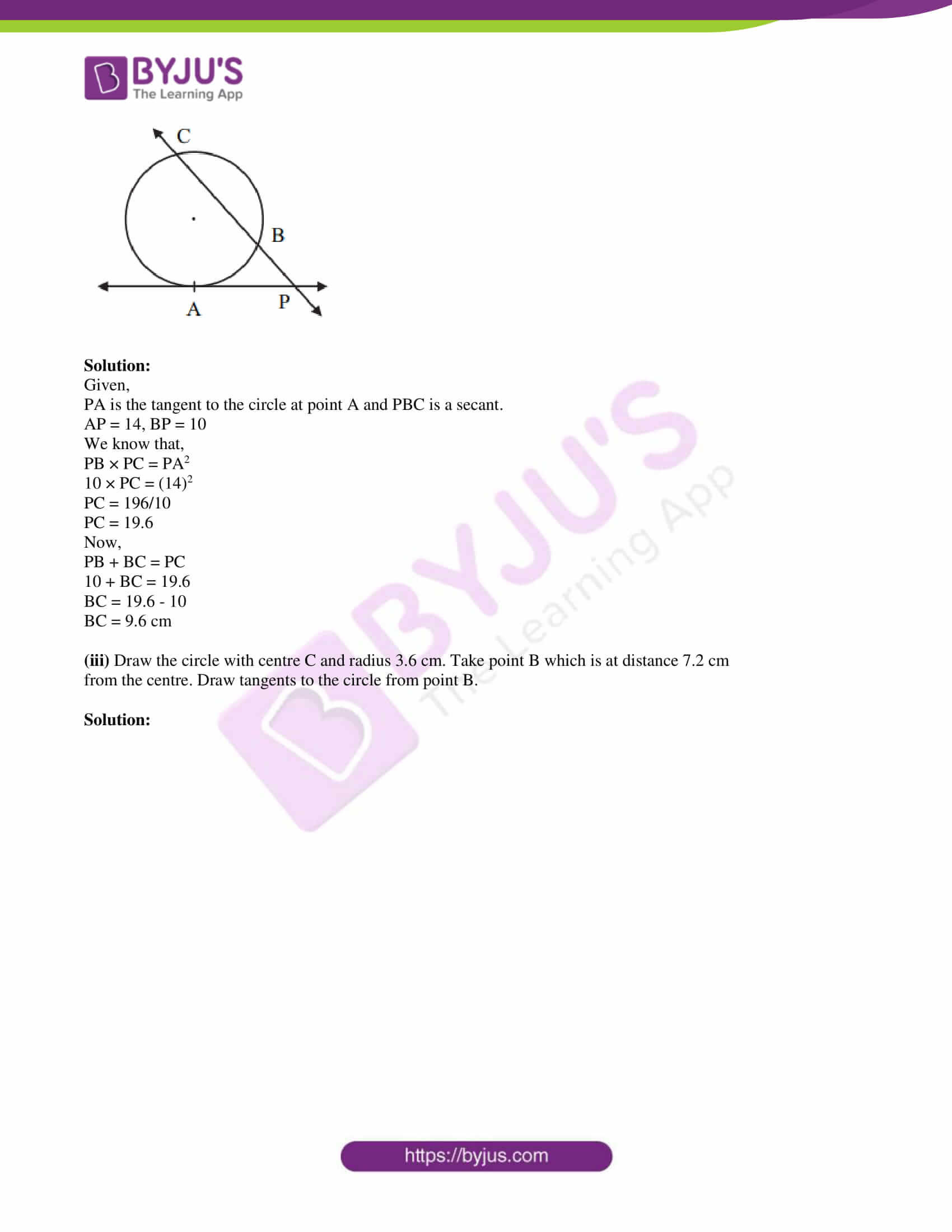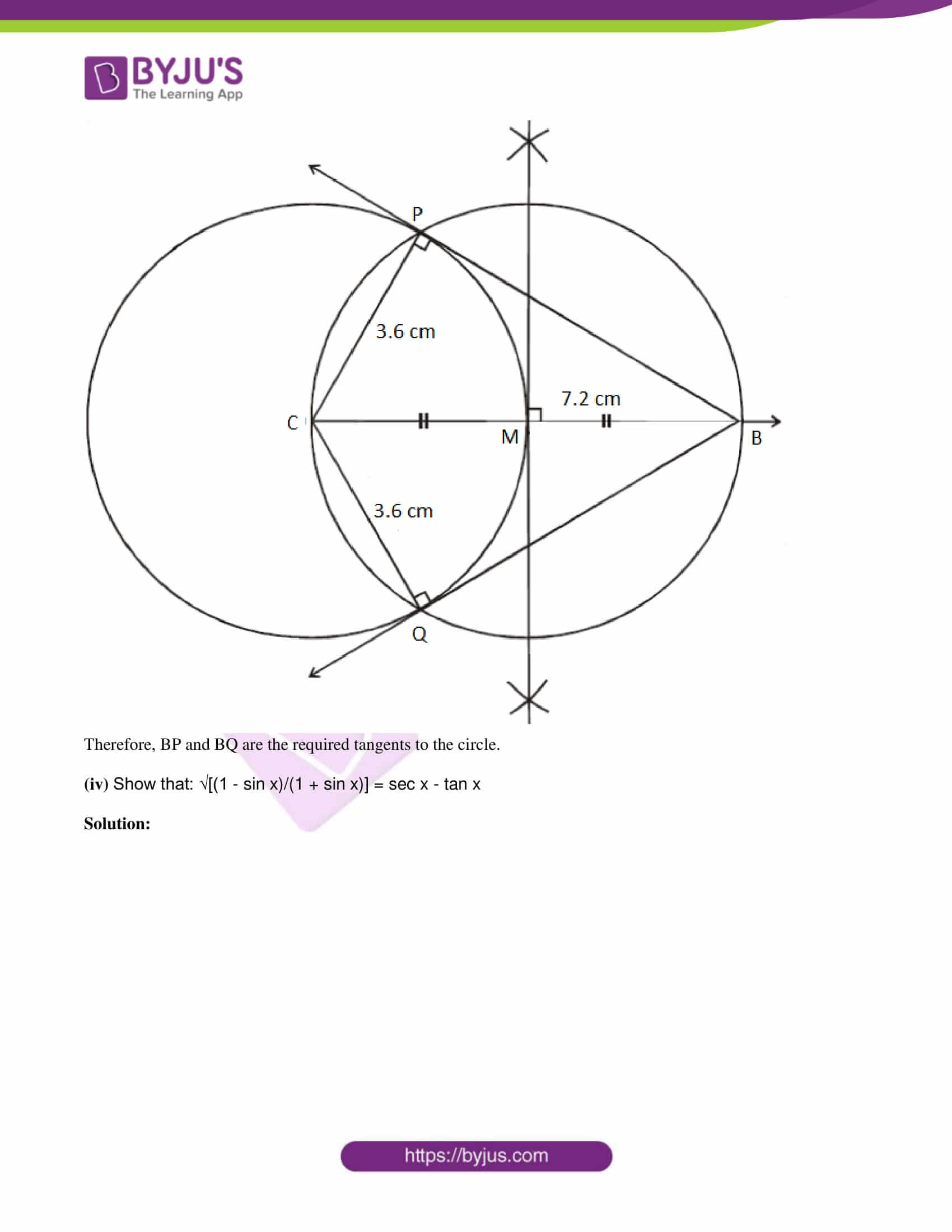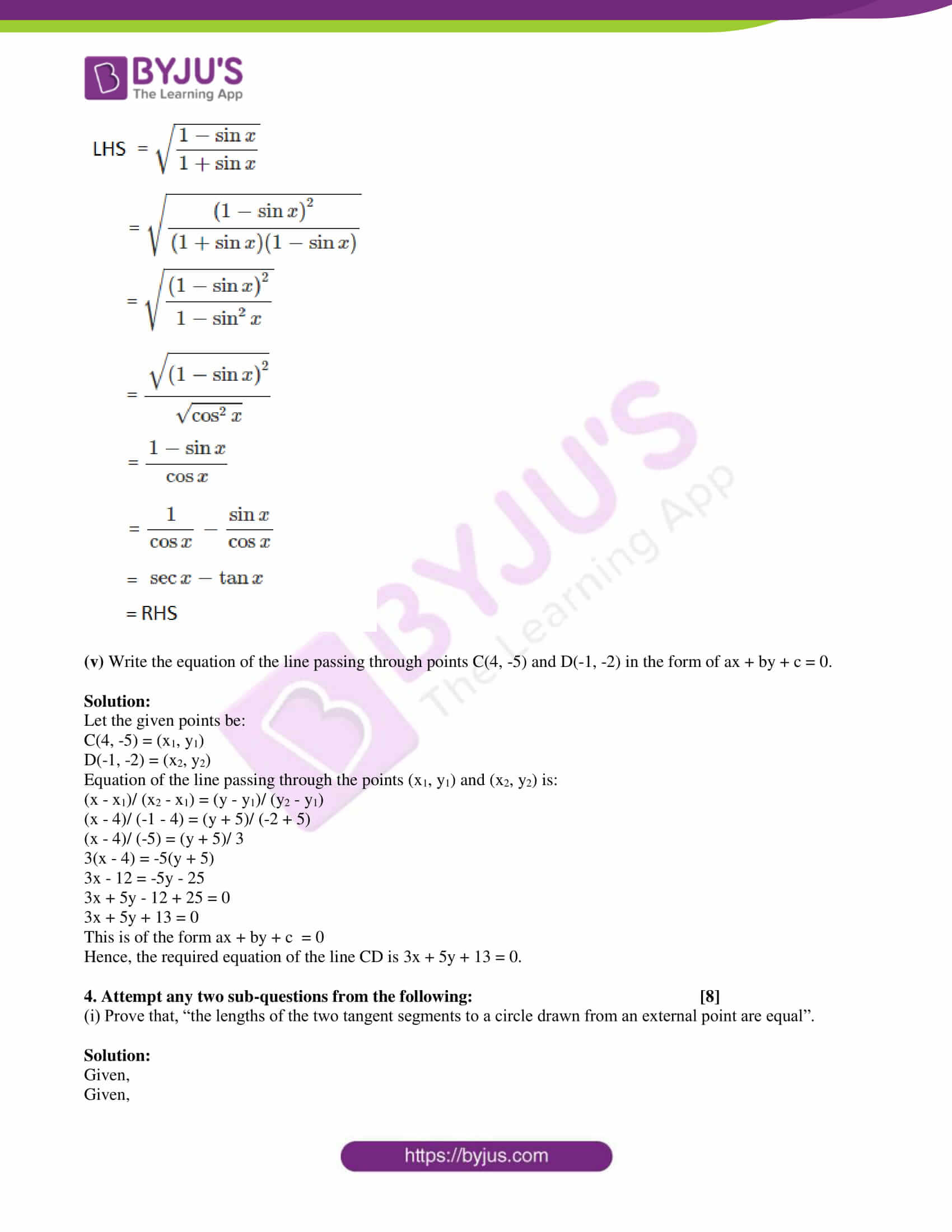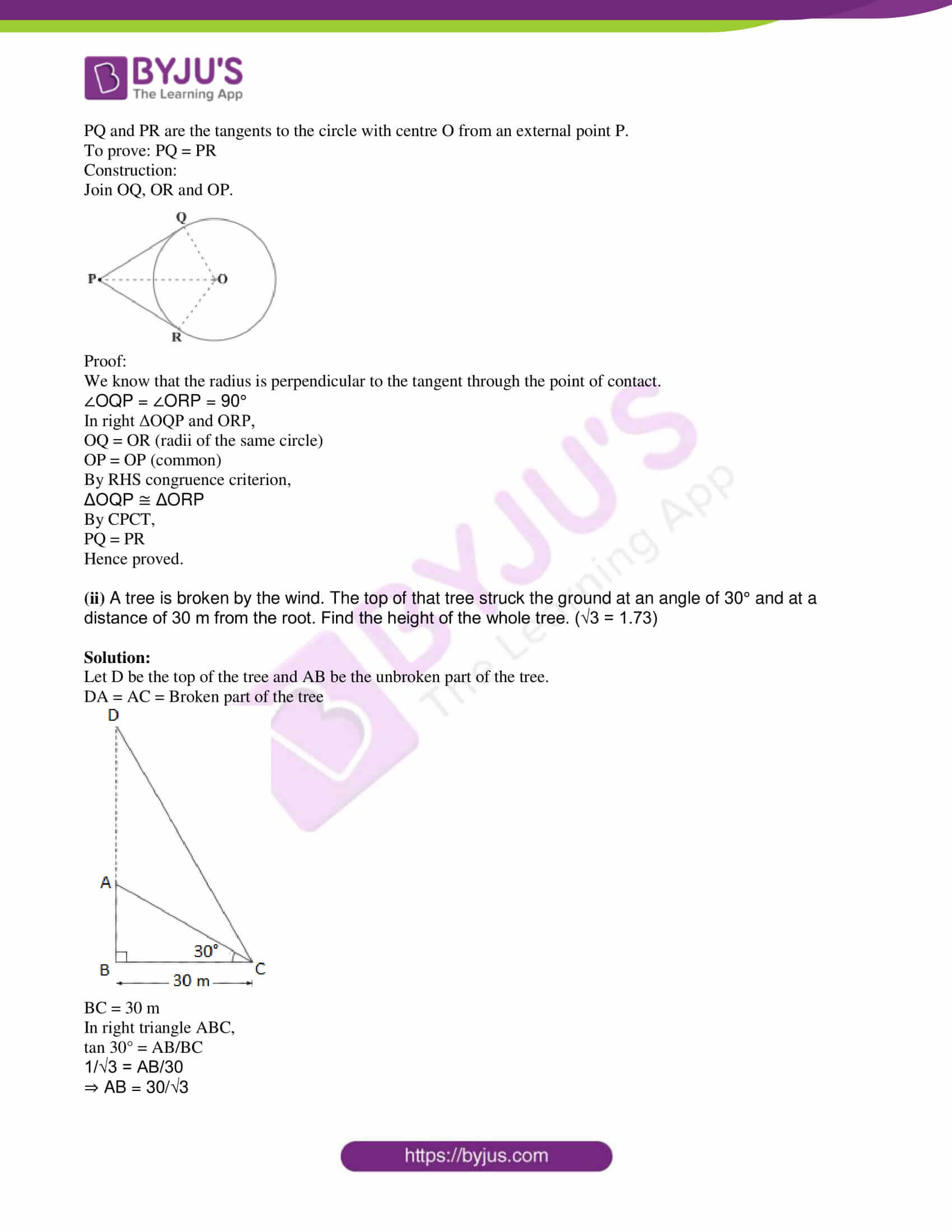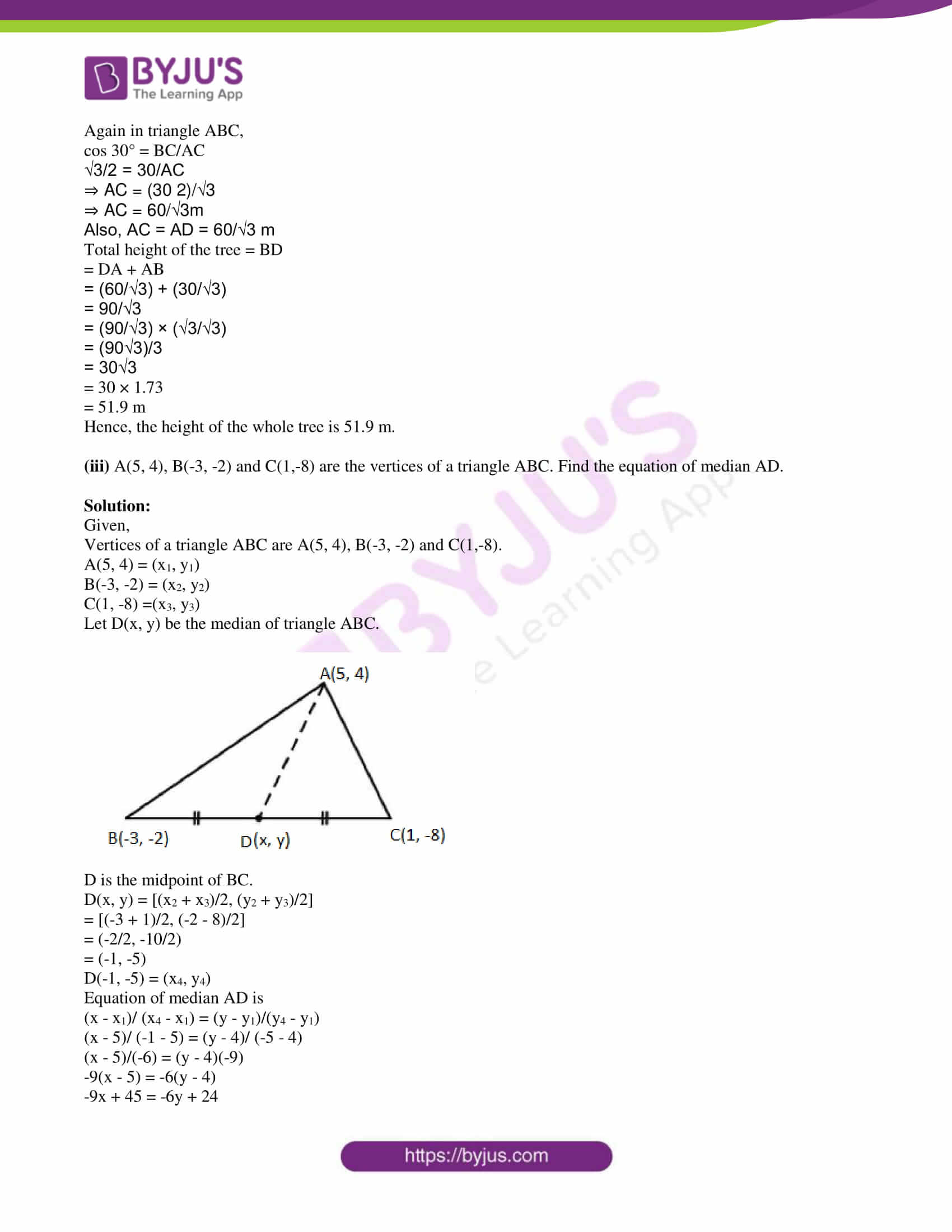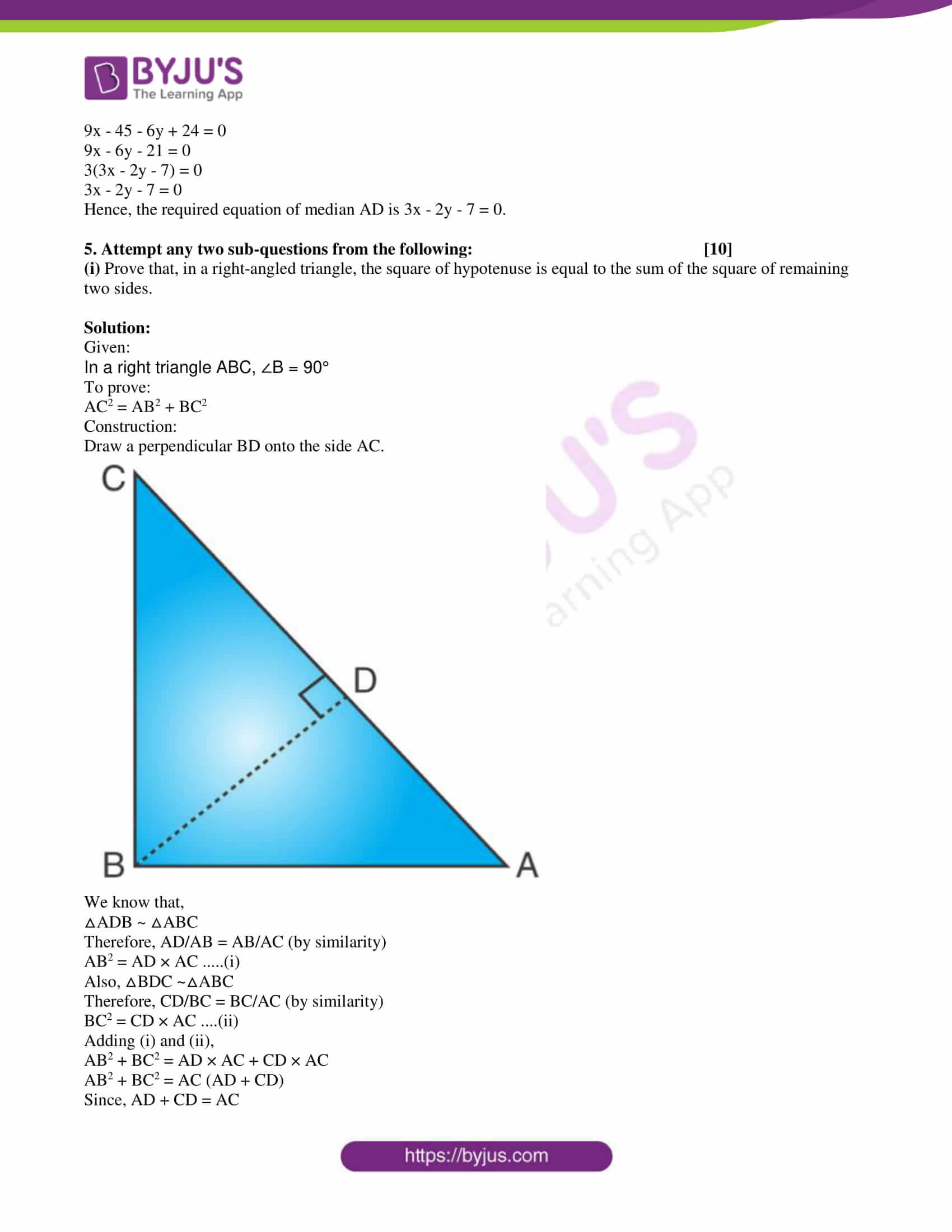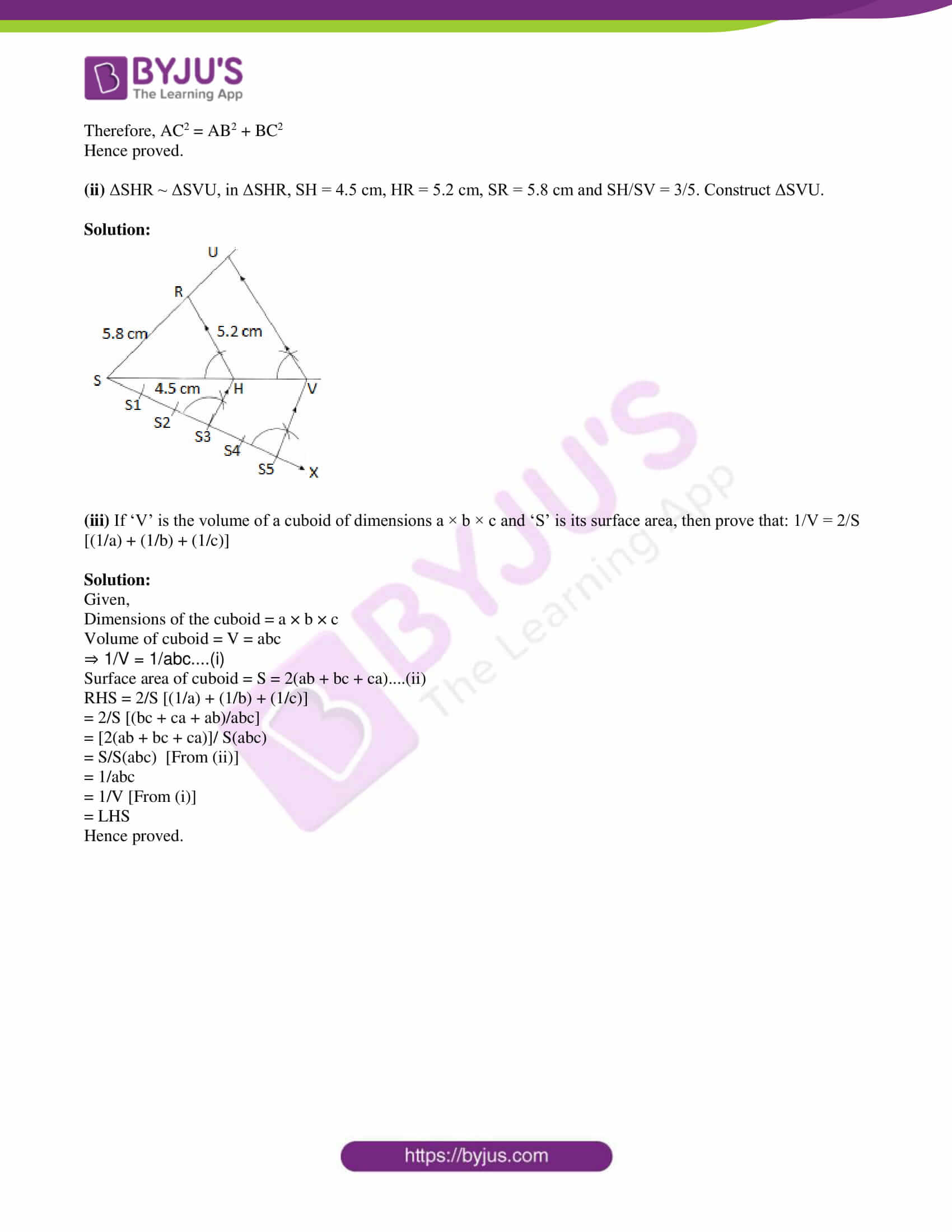PART – A

1. Attempt any five sub-questions from the following: 

(i) ΔDEF ~ ΔMNK. If DE = 5 and MN = 6, then find the value of A(ΔDEF)/ A(ΔMNK).

Solution:

Given,

ΔDEF ~ ΔMNK

DE = 5, MN = 6

We know that the ratio of areas of similar triangles is equal to squares of ratio of their corresponding sides.

A(ΔDEF)/ A(ΔMNK) = DE2/MN2

= (5)2/(6)2

= 25/36

(ii) If two circles with radii 8 cm and 3 cm respectively touch externally, then find the distance between their centres.

Solution:

Given,

Two circles with radii 8 cm and 3 cm respectively touch externally.

Distance between their centre = Sum of the radii

= 8 + 3

= 11 cm

(iii) Find the length of the altitude of an equilateral triangle with side 6 cm.

Solution:

Let ABC be an equilateral triangle with side 6 cm.

AD be the altitude of triangle ABC.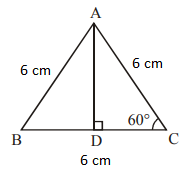⇒ AD = (√3/2) × 6

= 3√3 cm

Alternative method:

Altitude of an equilateral triangle = (√3/2) × side

= (√3/2) × 6

= 3√3 cm

(iv) If θ = 45°, then find tan θ.

Solution:

Given,

θ = 45°

tan θ = tan 45° = 1

(v) Slope of a line is 3 and y intercept is -4. Write the equation of a line.

Solution:

Given,

Slope of a line = m = 3

y intercept = c = -4

Equation of line having slope m and y-intercept c is

y = mx + c

Hence, the required equation of line is y = 3x – 4.

(vi) Using Euler’s formula, find V, if E = 30, F = 12.

Solution:

Given,

E = 30, F = 12

Using Euler’s formula,

F + V = E + 2

12 + V = 30 + 2

V = 32 – 12

V = 20

2. Attempt any four sub-questions from the following: 

(i) The ratio of the areas of two triangles with common base is 4 : 3. Height of the larger triangle

is 6 cm, then find the corresponding height of the smaller triangle.

Solution:

Let H be the height of the larger triangle and h be the height of the smaller triangle.

We know that the ratio of the areas of two triangles with common base is equal to the ratio of their corresponding heights.

⇒ 4/3 = H/h

⇒ 4/3 = 6/h (given height of the larger triangle is 6 cm)

⇒ h = (6 × 3)/4

⇒ h = 9/2 = 4.5 cm

Hence, the corresponding height of the smaller triangle is 4.5 cm.

(ii) In the following figure, point ‘A’ is the centre of the circle. Line MN is tangent at point M. If AN = 12 cm and MN = 6 cm, determine the radius of the circle.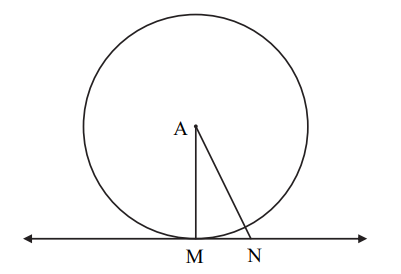Solution:

Given,

AN = 12 cm

MN = 6 cm

We know that the radius is perpendicular to the tangent through the point of contact.

Thus, ∠AMN = 90°

In right triangle AMN,

AN2 = AM2 + MN2

(12)2 = AM2 + (6)2

⇒ AM2 = 144 – 36

= 108

⇒ AM = √108 = 6√3

Hence, the radius of the circle is 6√3 cm.

(iii) Draw ∠PQR of measure 70° and bisect it.

Solution: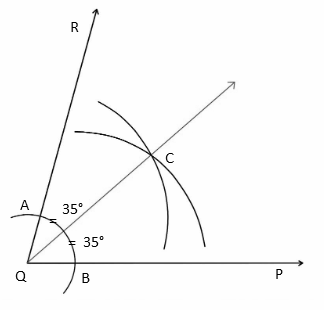Therefore, ∠PQR = 70° and QC is the bisector of it.

(iv) If cos θ = 3/5, where ‘θ’ is an acute angle. Find the value of sin θ.

Solution:

Given,

cos θ = ⅗

sin θ = √(1 – cos2θ)

= √[1 – (3/5)2]

= √[1 – (9/25)]

= √[(25 – 9)/25

= √(16/25)

= 4/5 (given that θ is an acute angle.

Therefore, sin θ = 4/5

(v) The volume of a cube is 1000 cm3. Find its side.

Solution:

Given,

Volume of cube = 1000 cm3

⇒ (side)3 = (10)3

⇒ Side = 10 cm

(vi) The radius and slant height of a cone are 4 cm and 25 cm respectively. Find the curved surface area of that cone. (π = 3.14)

Solution:

Given,

Radius of cone = r = 4 cm

Slant height = l = 25 cm

Curved surface area of cone = πrl

= 3.14 × 4 × 25

= 314 cm2

3. Attempt any three sub-questions from the following: 

(i) In the following figure, seg DH ⊥ seg EF and seg GK ⊥ seg EF. If DH = 6 cm, GK = 10 cm and A(ΔDEF) = 150 cm2, then find :

i. EF

ii. A( Δ GEF)

iii. A( Δ DFGE).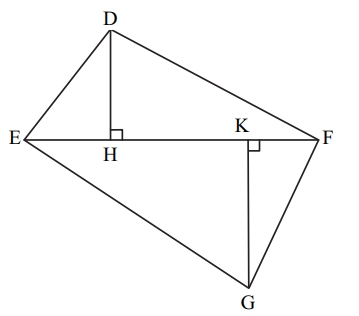Solution:

Given,

DH = 6 cm

GK = 10 cm

A(ΔDEF) = 150 cm2

i. A (ΔDEF) = (1/2) × EF × DH

150 = (1/2) × EF × 6

⇒ EF × 3 = 150

⇒ EF = 150/3

⇒ EF = 50 cm

ii. ΔDEF and ΔGEF have the common base EF.

Therefore, their areas are proportional to their corresponding heights.

A (ΔDEF)/ A (ΔGEF) = DH/GK

150/ A (ΔGEF) = 6/10

⇒ A (ΔGEF) = (150 × 10)/6 = 250

A (ΔGEF) = 250 cm2

iii. A (DFGE) = A (ΔDEF) + A (ΔGEF)

= 150 + 250

= 400 cm2

(ii) In the following figure, ray PA is the tangent to the circle at point A and PBC is a secant. If AP = 14, BP = 10, then find BC.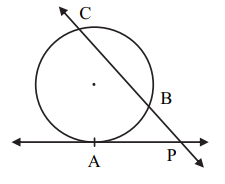Solution:

Given,

PA is the tangent to the circle at point A and PBC is a secant.

AP = 14, BP = 10

We know that,

PB × PC = PA2

10 × PC = (14)2

PC = 196/10

PC = 19.6

Now,

PB + BC = PC

10 + BC = 19.6

BC = 19.6 – 10

BC = 9.6 cm

(iii) Draw the circle with centre C and radius 3.6 cm. Take point B which is at distance 7.2 cm

from the centre. Draw tangents to the circle from point B.

Solution: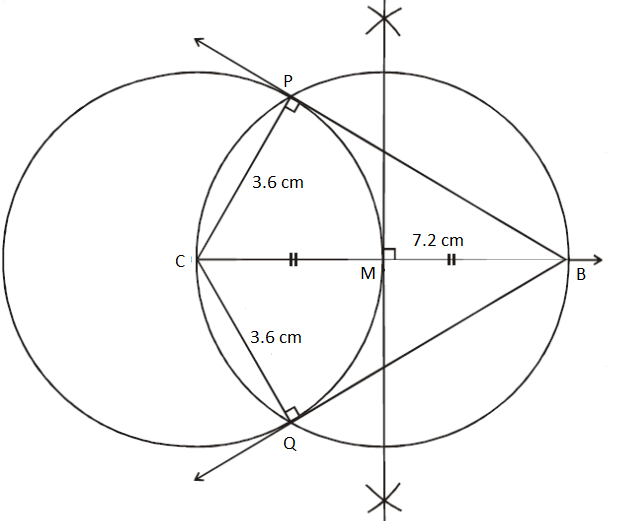Therefore, BP and BQ are the required tangents to the circle.

(iv) Show that: √[(1 – sin x)/(1 + sin x)] = sec x – tan x

Solution: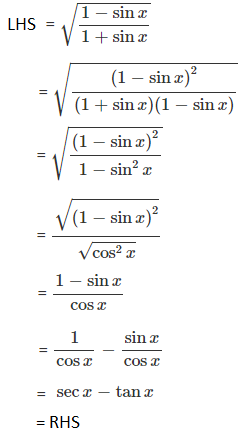(v) Write the equation of the line passing through points C(4, -5) and D(-1, -2) in the form of ax + by + c = 0.

Solution:

Let the given points be:

C(4, -5) = (x1, y1)

D(-1, -2) = (x2, y2)

Equation of the line passing through the points (x1, y1) and (x2, y2) is:

(x – x1)/ (x2 – x1) = (y – y1)/ (y2 – y1)

(x – 4)/ (-1 – 4) = (y + 5)/ (-2 + 5)

(x – 4)/ (-5) = (y + 5)/ 3

3(x – 4) = -5(y + 5)

3x – 12 = -5y – 25

3x + 5y – 12 + 25 = 0

3x + 5y + 13 = 0

This is of the form ax + by + c = 0

Hence, the required equation of the line CD is 3x + 5y + 13 = 0.

4. Attempt any two sub-questions from the following: 

(i) Prove that, “the lengths of the two tangent segments to a circle drawn from an external point are equal”.

Solution:

Given,

Given,

PQ and PR are the tangents to the circle with centre O from an external point P.

To prove: PQ = PR

Construction:

Join OQ, OR and OP.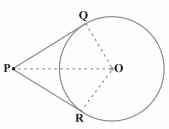Proof:

We know that the radius is perpendicular to the tangent through the point of contact.

∠OQP = ∠ORP = 90°

In right ΔOQP and ORP,

OQ = OR (radii of the same circle)

OP = OP (common)

By RHS congruence criterion,

ΔOQP ≅ ΔORP

By CPCT,

PQ = PR

Hence proved.

(ii) A tree is broken by the wind. The top of that tree struck the ground at an angle of 30° and at a distance of 30 m from the root. Find the height of the whole tree. (√3 = 1.73)

Solution:

Let D be the top of the tree and AB be the unbroken part of the tree.

DA = AC = Broken part of the tree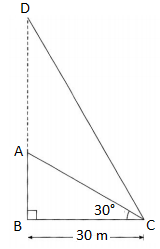BC = 30 m

In right triangle ABC,

tan 30° = AB/BC

1/√3 = AB/30

⇒ AB = 30/√3

Again in triangle ABC,

cos 30° = BC/AC

√3/2 = 30/AC

⇒ AC = (30 2)/√3

⇒ AC = 60/√3m

Also, AC = AD = 60/√3 m

Total height of the tree = BD

= DA + AB

= (60/√3) + (30/√3)

= 90/√3

= (90/√3) × (√3/√3)

= (90√3)/3

= 30√3

= 30 × 1.73

= 51.9 m

Hence, the height of the whole tree is 51.9 m.

(iii) A(5, 4), B(-3, -2) and C(1,-8) are the vertices of a triangle ABC. Find the equation of median AD.

Solution:

Given,

Vertices of a triangle ABC are A(5, 4), B(-3, -2) and C(1,-8).

A(5, 4) = (x1, y1)

B(-3, -2) = (x2, y2)

C(1, -8) =(x3, y3)

Let D(x, y) be the median of triangle ABC.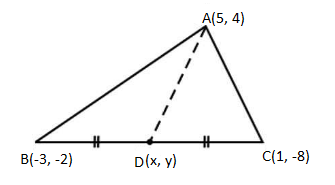D is the midpoint of BC.

D(x, y) = [(x2 + x3)/2, (y2 + y3)/2]

= [(-3 + 1)/2, (-2 – 8)/2]

= (-2/2, -10/2)

= (-1, -5)

D(-1, -5) = (x4, y4)

(x – x1)/ (x4 – x1) = (y – y1)/(y4 – y1)

(x – 5)/ (-1 – 5) = (y – 4)/ (-5 – 4)

(x – 5)/(-6) = (y – 4)(-9)

-9(x – 5) = -6(y – 4)

-9x + 45 = -6y + 24

9x – 45 – 6y + 24 = 0

9x – 6y – 21 = 0

3(3x – 2y – 7) = 0

3x – 2y – 7 = 0

Hence, the required equation of median AD is 3x – 2y – 7 = 0.

5. Attempt any two sub-questions from the following: 

(i) Prove that, in a right-angled triangle, the square of hypotenuse is equal to the sum of the square of remaining two sides.

Solution:

Given:

In a right triangle ABC, ∠B = 90°

To prove:

AC2 = AB2 + BC2

Construction:

Draw a perpendicular BD onto the side AC.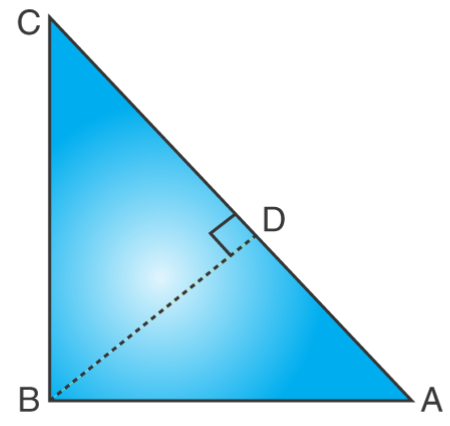We know that,

Therefore, AD/AB = AB/AC (by similarity)

AB2 = AD × AC …..(i)

Also, △BDC ~△ABC

Therefore, CD/BC = BC/AC (by similarity)

BC2 = CD × AC ….(ii)

AB2 + BC2 = AD × AC + CD × AC

AB2 + BC2 = AC (AD + CD)

Since, AD + CD = AC

Therefore, AC2 = AB2 + BC2

Hence proved.

(ii) ΔSHR ~ ΔSVU, in ΔSHR, SH = 4.5 cm, HR = 5.2 cm, SR = 5.8 cm and SH/SV = 3/5. Construct ΔSVU.

Solution: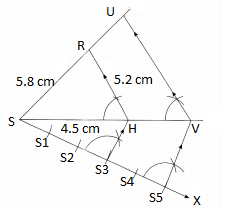(iii) If ‘V’ is the volume of a cuboid of dimensions a × b × c and ‘S’ is its surface area, then prove that: 1/V = 2/S [(1/a) + (1/b) + (1/c)]

Solution:

Given,

Dimensions of the cuboid = a × b × c

Volume of cuboid = V = abc

⇒ 1/V = 1/abc….(i)

Surface area of cuboid = S = 2(ab + bc + ca)….(ii)

RHS = 2/S [(1/a) + (1/b) + (1/c)]

= 2/S [(bc + ca + ab)/abc]

= [2(ab + bc + ca)]/ S(abc)

= S/S(abc) [From (ii)]

= 1/abc

= 1/V [From (i)]

= LHS

Hence proved.